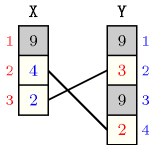## 5.202. inverse_within_range

Origin
Constraint

$\mathrm{𝚒𝚗𝚟𝚎𝚛𝚜𝚎}_\mathrm{𝚠𝚒𝚝𝚑𝚒𝚗}_\mathrm{𝚛𝚊𝚗𝚐𝚎}\left(𝚇,𝚈\right)$

Synonyms

$\mathrm{𝚒𝚗𝚟𝚎𝚛𝚜𝚎}_\mathrm{𝚒𝚗}_\mathrm{𝚛𝚊𝚗𝚐𝚎}$, $\mathrm{𝚒𝚗𝚟𝚎𝚛𝚜𝚎}_\mathrm{𝚛𝚊𝚗𝚐𝚎}$.

Arguments
 $𝚇$ $\mathrm{𝚌𝚘𝚕𝚕𝚎𝚌𝚝𝚒𝚘𝚗}\left(\mathrm{𝚟𝚊𝚛}-\mathrm{𝚍𝚟𝚊𝚛}\right)$ $𝚈$ $\mathrm{𝚌𝚘𝚕𝚕𝚎𝚌𝚝𝚒𝚘𝚗}\left(\mathrm{𝚟𝚊𝚛}-\mathrm{𝚍𝚟𝚊𝚛}\right)$
Restrictions
 $\mathrm{𝚛𝚎𝚚𝚞𝚒𝚛𝚎𝚍}$$\left(𝚇,\mathrm{𝚟𝚊𝚛}\right)$ $\mathrm{𝚛𝚎𝚚𝚞𝚒𝚛𝚎𝚍}$$\left(𝚈,\mathrm{𝚟𝚊𝚛}\right)$
Purpose

If the ${i}^{th}$ variable of the collection $𝚇$ is assigned to $j$ and if $j$ is greater than or equal to 1 and less than or equal to the number of items of the collection $𝚈$ then the ${j}^{th}$ variable of the collection $𝚈$ is assigned to $i$.

Conversely, if the ${j}^{th}$ variable of the collection $𝚈$ is assigned to $i$ and if $i$ is greater than or equal to 1 and less than or equal to the number of items of the collection $𝚇$ then the ${i}^{th}$ variable of the collection $𝚇$ is assigned to $j$.

Example
$\left(〈9,4,2〉,〈9,3,9,2〉\right)$

Since the second item of $𝚇$ is assigned to 4, the fourth item of $𝚈$ is assigned to 2. Similarly, since the third item of $𝚇$ is assigned to 2, the second item of $𝚈$ is assigned to 3. Figure 5.202.1 illustrates the correspondence between $𝚇$ and $𝚈$.

##### Figure 5.202.1. Correspondence between the items of $𝚇=〈9,4,2〉$ and the items of $𝚈=〈9,3,9,2〉$: on the $𝚇$ side values between 1 and $|𝚈|=4$ are shown in blue, on the $𝚈$ side values between 1 and $|𝚇|=3$ are shown in red.Typical
 $|𝚇|>1$ $\mathrm{𝚛𝚊𝚗𝚐𝚎}$$\left(𝚇.\mathrm{𝚟𝚊𝚛}\right)>1$ $|𝚈|>1$ $\mathrm{𝚛𝚊𝚗𝚐𝚎}$$\left(𝚈.\mathrm{𝚟𝚊𝚛}\right)>1$
Symmetry

Arguments are permutable w.r.t. permutation $\left(𝚇,𝚈\right)$.

Usage

Consider an integer value $m$ and a sequence of $n$ variables $S$ from which you have to select a subsequence ${S}^{\text{'}}$ such that:

• All variables of ${S}^{\text{'}}$ have to be assigned to distinct values from $\left[1,m\right]$,

• All variables not in ${S}^{\text{'}}$ have to be assigned a value, not necessarily distinct, outside $\left[1,m\right]$.

As for the $\mathrm{𝚒𝚗𝚟𝚎𝚛𝚜𝚎}$ constraint we may want to create explicitly a value variable for each value in [1,m] in order to state some specific constraints on the value variables or to use a heuristics involving the original variables of $S$ as well as the value variables. The purpose of the $\mathrm{𝚒𝚗𝚟𝚎𝚛𝚜𝚎}_\mathrm{𝚠𝚒𝚝𝚑𝚒𝚗}_\mathrm{𝚛𝚊𝚗𝚐𝚎}$ constraint is to link the variables of $S$ with the value variables.

specialisation: $\mathrm{𝚒𝚗𝚟𝚎𝚛𝚜𝚎}$ (the 2 collections have not necessarly the same number of items).

Keywords
Arc input(s)

$𝚇$ $𝚈$

Arc generator
$\mathrm{𝑆𝑌𝑀𝑀𝐸𝑇𝑅𝐼𝐶}_\mathrm{𝑃𝑅𝑂𝐷𝑈𝐶𝑇}$$↦\mathrm{𝚌𝚘𝚕𝚕𝚎𝚌𝚝𝚒𝚘𝚗}\left(𝚜\mathtt{1},𝚜\mathtt{2}\right)$

Arc arity
Arc constraint(s)
$𝚜\mathtt{1}.\mathrm{𝚟𝚊𝚛}=𝚜\mathtt{2}.\mathrm{𝚔𝚎𝚢}$
Graph class
 $•$$\mathrm{𝙱𝙸𝙿𝙰𝚁𝚃𝙸𝚃𝙴}$ $•$$\mathrm{𝙽𝙾}_\mathrm{𝙻𝙾𝙾𝙿}$ $•$$\mathrm{𝚂𝚈𝙼𝙼𝙴𝚃𝚁𝙸𝙲}$